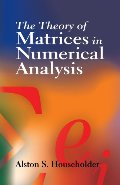# libib

## Theory of Matrices in Numerical Analysis (Dover Books on Mathematics), TheAlston S. Householder, Mathematics
This text explores aspects of matrix theory that are most useful in developing and appraising computational methods for solving systems of linear equations and for finding characteristic roots. Suitable for advanced undergraduates and graduate students, it assumes an understanding of the general principles of matrix algebra, including the Cayley-Hamilton theorem, characteristic roots and vectors, and linear dependence.An introductory chapter covers the Lanczos algorithm, orthogonal polynomials, and determinantal identities. Succeeding chapters examine norms, bounds, and convergence; localization theorems and other inequalities; and methods of solving systems of linear equations. The final chapters illustrate the mathematical principles underlying linear equations and their interrelationships. Topics include methods of successive approximation, direct methods of inversion, normalization and reduction of the matrix, and proper values and vectors. Each chapter concludes with a helpful set of references and problems.

No reviews
Item Posts
No posts